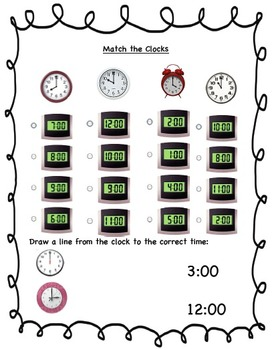Math Practice or Homework WorksheetsSubject
Resource Type
Product Rating
4.0
2 Ratings
File Type

PDF (Acrobat) Document File

Be sure that you have an application to open this file type before downloading and/or purchasing.

564 KB|4 pages
Share
Product Description
Adding ten more and ten less:
CCSS.Math.Content.1.NBT.C.5 Given a two-digit number, mentally find 10 more or 10 less than the number, without having to count; explain the reasoning used.

CCSS.Math.Content.1.OA.C.6 Add and subtract within 20, demonstrating fluency for addition and subtraction within 10. Use strategies such as counting on; making ten (e.g., 8 + 6 = 8 + 2 + 4 = 10 + 4 = 14); decomposing a number leading to a ten (e.g., 13 – 4 = 13 – 3 – 1 = 10 – 1 = 9); using the relationship between addition and subtraction (e.g., knowing that 8 + 4 = 12, one knows 12 – 8 = 4); and creating equivalent but easier or known sums (e.g., adding 6 + 7 by creating the known equivalent 6 + 6 + 1 = 12 + 1 = 13).

Using clocks to tell time:
CCSS.Math.Content.1.MD.B.3 Tell and write time in hours and half-hours using analog and digital clocks.

Identifying Patterns and Continuing a Pattern
Total Pages
4 pages
N/A
Teaching Duration
N/A
Report this Resource to TpT
Reported resources will be reviewed by our team. Report this resource to let us know if this resource violates TpT’s content guidelines.